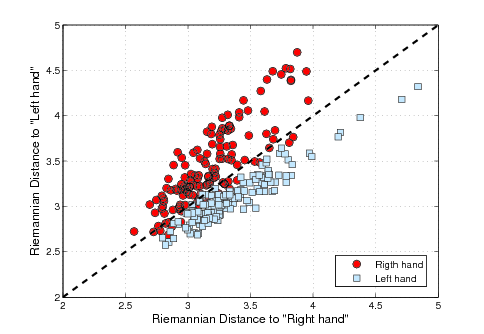This paper presents a new classification framework for brain-computer interface (BCI) based on motor imagery. This framework involves the concept of Riemannian geometry in the manifold of covariance matrices. The main idea is to use spatial covariance matrices as EEG signal descriptors and to rely on Riemannian geometry to directly classify these matrices using the topology of the manifold of symmetric and positive definite (SPD) matrices. This framework allows to extract the spatial information contained in EEG signals without using spatial filtering. Two methods are proposed and compared with a reference method [multiclass Common Spatial Pattern (CSP) and Linear Discriminant Analysis (LDA)] on the multiclass dataset IIa from the BCI Competition IV. The first method, named minimum distance to Riemannian mean (MDRM), is an implementation of the minimum distance to mean (MDM) classification algorithm using Riemannian distance and Riemannian mean. This simple method shows comparable results with the reference method. The second method, named tangent space LDA (TSLDA), maps the covariance matrices onto the Riemannian tangent space where matrices can be vectorized and treated as Euclidean objects. Then, a variable selection procedure is applied in order to decrease dimensionality and a classification by LDA is performed. This latter method outperforms the reference method increasing the mean classification accuracy from 65.1% to 70.2%.